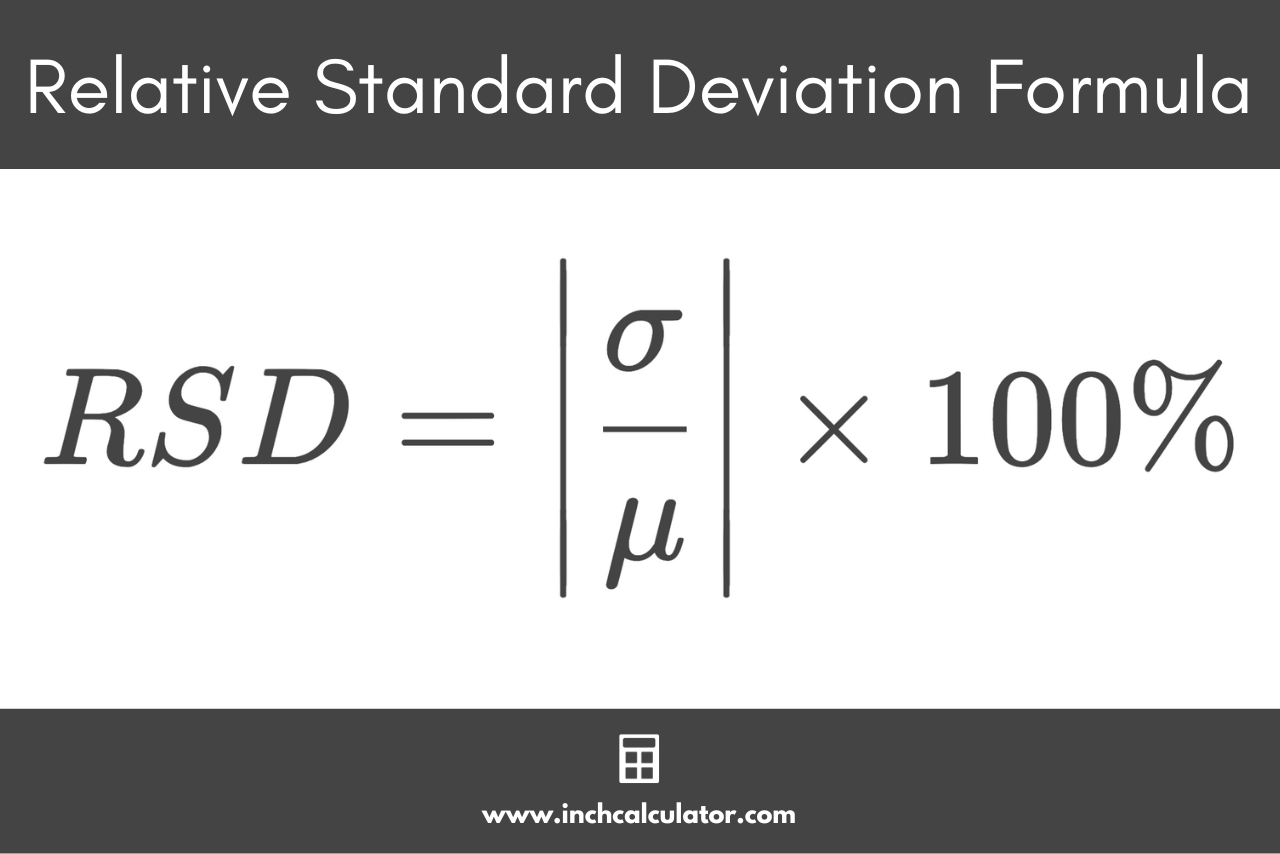# Relative Standard Deviation Calculator

Enter a data set or the standard deviation and mean if known to calculate relative standard deviation using the calculator below.

Separate numbers using a comma (,)

## Results:

Learn how we calculated this below

## How to Calculate Relative Standard Deviation

Relative standard deviation, or RSD, is a measure of how closely the data is clustered around the mean in a sample or population. It provides an intuitive way of comparing variability across groups in terms of percentages, where comparisons would otherwise be difficult.

For example, men’s organs are larger on average than women’s, but also more variable, and the RSD can be used to quantify such differences.

The relative standard deviation is a statistical measure that is equal to the absolute value of the coefficient of variation, which is a ratio of the standard deviation to the mean.

Because it’s an absolute value, the RSD will always be positive, and it will never be a negative value.

### Relative Standard Deviation Formula

Because the relative standard deviation is the absolute value of the coefficient of variation, you can take the absolute value of the CV formula to find it.

Thus, the formula to calculate the relative standard deviation (RSD) is:

RSD=\left |\frac{\sigma}{\mu}\right |

Where:
σ = standard deviation
μ = mean

The formula states that the relative standard deviation RSD is equal to the absolute value of the standard deviation σ (pronounced sigma) divided by the mean μ (pronounced mu).

The relative standard deviation can also be expressed as a percentage. To express RSD in percent form, multiply by 100, then add a percent symbol (%).

RSD=\left |\frac{\sigma}{\mu}\right | \times 100\%For example, let’s calculate the relative standard deviation given a standard deviation of 1.2 and a mean of 14.6.

RSD=\left |\frac{1.2}{14.6}\right |
RSD=0.082

In this example, the relative standard deviation is equal to 0.082.

To express RSD as a percentage, multiply it by 100.

0.082 × 100 = 8.2

In this example, the relative standard deviation is equal to 0.082 or 8.2%.

Try our statistics calculator for more measurements of a dataset.